Contents | Prev | Next | Index

# 21.9 The Class `java.util.Random`

Each instance of class `Random` serves as a separate, independent pseudorandom generator of primitive values.

```public class `Random` {
protected long `seed`;
protected double `nextNextGaussian`;
protected boolean `haveNextNextGaussian` = false;
public `Random`();
public `Random`(long seed);
public void `setSeed`(long seed);
protected int `next`(int bits);
public int `nextInt`();
public long `nextLong`();
public float `nextFloat`();
public double `nextDouble`();
public double `nextGaussian`();
}
```
If two `Random` objects are created with the same seed and the same sequence of method calls is made for each, they will generate and return identical sequences of numbers in all Java implementations. In order to guarantee this property, particular algorithms are specified for the class `Random`. Java implementations must use all the algorithms shown here for the class `Random`, for the sake of absolute portability of Java code. However, subclasses of class `Random` are permitted use other algorithms, so long as they adhere to the general contracts for all the methods.

The algorithms implemented by class `Random` use three state variables, which are `protected`. They also use a `protected` utility method that on each invocation can supply up to up to 32 pseudorandomly generated bits.

21.9.1 `protected long seed;`

A variable used by method `next` (§21.9.7) to hold the state of the pseudorandom number generator.

21.9.2 `protected double nextNextGaussian;`

A variable used by method `nextGaussian` (§21.9.12) to hold a precomputed value to be delivered by that method the next time it is called.

21.9.3 `protected boolean haveNextNextGaussian = false;`

A variable used by method `nextGaussian` (§21.9.12) to keep track of whether it has precomputed and stashed away the next value to be delivered by that method.

21.9.4 `public Random()`

This constructor initializes a newly created `Random` number generator by using the current time of day (§20.18.6) as a seed.

```public Random() { this(System.currentTimeMillis()); }
```

21.9.5 `public Random(long seed)`

This constructor initializes a newly created `Random` number generator by using the argument `seed` as a seed.

```
public Random(long seed) { setSeed(seed); }
```

21.9.6 `public void setSeed(long seed)`

The general contract of `setSeed` is that it alters the state of this random number generator object so as to be in exactly the same state as if it had just been created with the argument `seed` as a seed.

The method `setSeed` is implemented by class `Random` as follows:

```
synchronized public void setSeed(long seed) {
this.seed = (seed ^ 0x5DEECE66DL) & ((1L << 48) - 1);
haveNextNextGaussian = false;
}
```
The implementation of `setSeed` by class `Random` happens to use only 48 bits of the given seed. In general, however, an overriding method may use all 64 bits of the long argument as a seed value.

[In certain early versions of Java, the `setSeed` method failed to reset the value of `haveNextNextGaussian` to `false`; this flaw could lead to failure to produce repeatable behavior.]

21.9.7 `protected int next(int bits)`

The general contract of `next` is that it returns an `int` value and if the argument bits is between `1` and `32` (inclusive), then that many low-order bits of the returned value will be (approximately) independently chosen bit values, each of which is (approximately) equally likely to be `0` or `1`.

The method `next` is implemented by class `Random` as follows:

```
synchronized protected int next(int bits) {
seed = (seed * 0x5DEECE66DL + 0xBL) & ((1L << 48) - 1);
return (int)(seed >>> (48 - bits));
}
```
This is a linear congruential pseudorandom number generator, as defined by D. H. Lehmer and described by Donald E. Knuth in The Art of Computer Programming, Volume 2: Seminumerical Algorithms, section 3.2.1.

21.9.8 `public int nextInt()`

The general contract of `nextInt` is that one `int` value is pseudorandomly generated and returned. All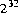possible `int` values are produced with (approximately) equal probability.

The method `setSeed` is implemented by class `Random` as follows:

```public int nextInt() {  return next(32); }
```

21.9.9 `public long nextLong()`

The general contract of `nextLong` is that one `long` value is pseudorandomly generated and returned. All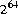possible `long` values are produced with (approximately) equal probability.

The method `setSeed` is implemented by class `Random` as follows:

```
public long nextLong() {
return ((long)next(32) << 32) + next(32);
}
```

21.9.10 `public float nextFloat()`

The general contract of `nextFloat` is that one `float` value, chosen (approximately) uniformly from the range `0.0f` (inclusive) to `1.0f` (exclusive), is pseudorandomly generated and returned. All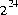possible `float` values of the form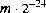, where m is a positive integer less than, are produced with (approximately) equal probability.

The method `setSeed` is implemented by class `Random` as follows:

```
public float nextFloat() {
return next(24) / ((float)(1 << 24));
}
```
The hedge "approximately" is used in the foregoing description only because the `next` method is only approximately an unbiased source of independently chosen bits. If it were a perfect source or randomly chosen bits, then the algorithm shown would choose `float` values from the stated range with perfect uniformity.

[In early versions of Java, the result was incorrectly calculated as:

```	return next(30) / ((float)(1 << 30));
```
This might seem to be equivalent, if not better, but in fact it introduced a slight nonuniformity because of the bias in the rounding of floating-point numbers: it was slightly more likely that the low-order bit of the significand would be `0` than that it would be `1`.]

21.9.11 `public double nextDouble()`

The general contract of `nextDouble` is that one `double` value, chosen (approximately) uniformly from the range `0.0d` (inclusive) to `1.0d` (exclusive), is pseudorandomly generated and returned. All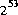possible `float` values of the form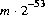, where m is a positive integer less than, are produced with (approximately) equal probability.

The method `setSeed` is implemented by class `Random` as follows:

```
public double nextDouble() {
return (((long)next(26) << 27) + next(27))
/ (double)(1L << 53);
}
```
The hedge "approximately" is used in the foregoing description only because the `next` method is only approximately an unbiased source of independently chosen bits. If it were a perfect source or randomly chosen bits, then the algorithm shown would choose `double` values from the stated range with perfect uniformity.

[In early versions of Java, the result was incorrectly calculated as:

```
return (((long)next(27) << 27) + next(27))
/ (double)(1L << 54);
```
This might seem to be equivalent, if not better, but in fact it introduced a large nonuniformity because of the bias in the rounding of floating-point numbers: it was three times as likely that the low-order bit of the significand would be `0` than that it would be `1`! This nonuniformity probably doesn't matter much in practice, but we strive for perfection.]

21.9.12 `public double nextGaussian()`

The general contract of `nextGaussian` is that one `double` value, chosen from (approximately) the usual normal distribution with mean `0.0` and standard deviation `1.0`, is pseudorandomly generated and returned.

The method `setSeed` is implemented by class `Random` as follows:

```
synchronized public double nextGaussian() {
if (haveNextNextGaussian) {
haveNextNextGaussian = false;
return nextNextGaussian;
} else {
double v1, v2, s;
do {
v1 = 2 * nextDouble() - 1; 	// between -1.0 and 1.0
v2 = 2 * nextDouble() - 1; 	// between -1.0 and 1.0
s = v1 * v1 + v2 * v2;
} while (s >= 1);
double norm = Math.sqrt(-2 * Math.log(s)/s);
nextNextGaussian = v2 * norm;
haveNextNextGaussian = true;
return v1 * norm;
}
}
```
This uses the polar method of G. E. P. Box, M. E. Muller, and G. Marsaglia, as described by Donald E. Knuth in The Art of Computer Programming, Volume 2: Seminumerical Algorithms, section 3.4.1, subsection C, algorithm P. Note that it generates two independent values at the cost of only one call to `Math.log` and one call to `Math.sqrt`.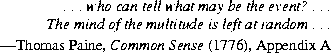Java Language Specification (HTML generated by Suzette Pelouch on February 24, 1998)